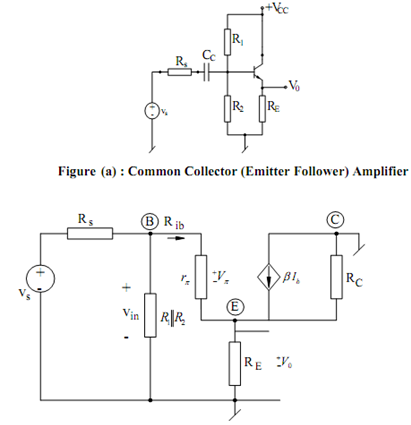## Common-collector Amplifier Assignment Help

Assignment Help: >> Transistor Amplifier Circuits - Common-collector Amplifier

Common-collector (Emitter Follower) Amplifier:

The basic emitter follower arrangement is illustrated in Figure (a), while its small signal ac equivalent circuit is illustrated in Figure (b).Figure (b) : Small Signal Equivalent of Common Collector Amplifier

From the circuit, we see that I0 = (1 + β) ib so that the output voltage V0 may be written as

V0  = (1 + β) ib  (r0  // RE )

The loop equation of the base-emitter loop is

Vin  = ib [rπ + (1 + β) (r0  // RE )

from where we get :

Rib = Vin/ ib=  rπ + (1 + β) (r0  // RE )

Furthermore,

Vin  = (Ri / Ri  + Rs)Vs  where  Ri  = R1 // R2  // Rib

From the above equations, at last we find

A  = V0  / V ( (1 + β) [r0  // RE ] /  rπ  + (1 + β) (ro  // RE ))(Ri/ Ri  + Rs )

Therefore, it is seen that gain is positive which means that output and input are in same phase.

Also, if (1 + β) (r0 // RE) >> rπ and Ri >> Rs

Av ≅ 1 (In practice, it is, though always slightly less than unity). The small signal output resistance of the circuit is specified by

R0  =     ( rπ + (R1  || R2  || R3 ) /(1 + β))|| RE|| r0

From the equivalent circuit, it follows that for Vs = 0 (or Vπ = 0), the small signal resistance looking into the output terminal shall be equal to Rc, Thus,

R0 = RC